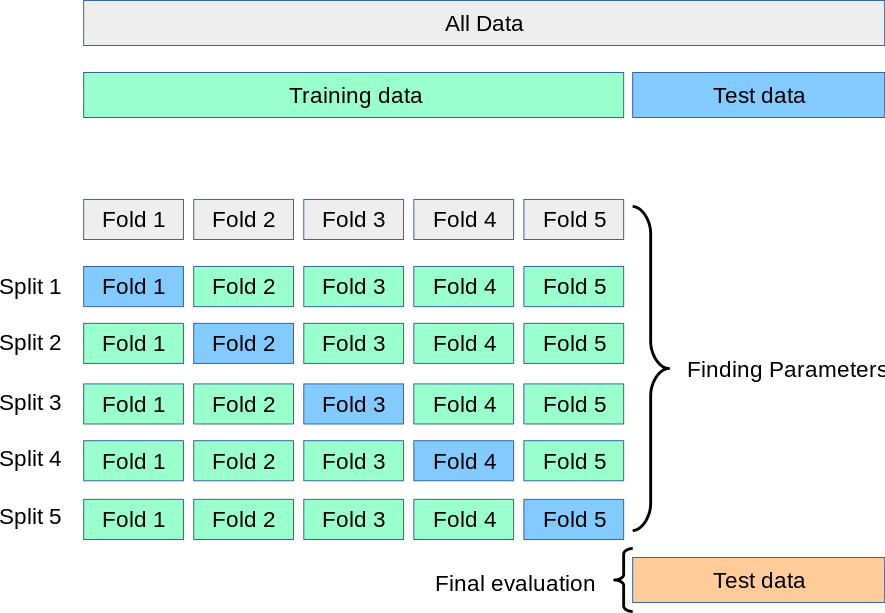You want to score a list of models with cross-validation with customized scoring methods.

## Why Cross-validation?⌗

A common approach to machine learning is to split your data into three different sets: a training set, a test set, and a validation set.

Learning the parameters of a prediction function and testing it on the same data is a methodological mistake: a model that would just repeat the labels of the samples that it has just seen would have a perfect score but would fail to predict anything useful on yet-unseen data.

[…], yet another part of the dataset can be held out as a so-called “validation set”: training proceeds on the training set, after which evaluation is done on the validation set, and when the experiment seems to be successful, final evaluation can be done on the test set. 1

That’s why we use cross-validation (CV). CS splits the data into smaller sets, and trains and evaluates the model repeatedly:image from sci-kit learn

## How to Create Cross-Validated Metrics⌗

The easies way to use cross-validation with sci-kit learn is the `cross_val_score` function.

The function uses the default scoring method for each model. For example, if you use Gaussian Naive Bayes, the scoring method is the mean accuracy on the given test data and labels.

### The Problem⌗

You have more than one model that you want to score. The default scoring parameters don’t work across all models, so you have to define your own metrics.

For example, you have a multi-class classification problem and want to score `f1`. `cross_val_score` returns:

``````ValueError: Target is multiclass but average='binary'. Please choose another average setting
``````

### The Solution⌗

Use `cross_validate` and specify the metrics you need. Create your own metrics with `make_score`.

1. Create a dictionary of scoring metrics:
``````scoring = {'accuracy': 'accuracy',
'precision': make_scorer(precision_score, average='weighted'),
'recall': make_scorer(recall_score, average='weighted'),
'f1': make_scorer(f1_score, average='weighted'),
'log_loss': 'neg_log_loss'
}
``````
1. Create a helper function for `cross_validate` that returns the average score:
``````def average_score_on_cross_val_classification(clf, X, y, scoring=scoring, cv=skf):
"""
Evaluates a given model/estimator using cross-validation
and returns a dict containing the absolute vlues of the average (mean) scores
for classification models.

clf: scikit-learn classification model
X: features (no labels)
y: labels
scoring: a dictionary of scoring metrics
cv: cross-validation strategy
"""
# Score metrics on cross-validated dataset
scores_dict = cross_validate(clf, X, y, scoring=scoring, cv=skf, n_jobs=-1)

# return the average scores for each metric
return {metric: round(np.mean(scores), 5) for metric, scores in scores_dict.items()}

``````
1. Use the custom function on a fitted model
``````average_score_on_cross_val_classification(naive_bayes_clf, X, y)

> {'fit_time': 0.33044,
>  'score_time': 0.21879,
>  'test_accuracy': 0.81145,
>  'test_precision': 0.82919,
>  'test_recall': 0.81145,
>  'test_f1': 0.80181,
>  'test_log loss': -2.13586}
``````